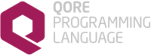Qore SqlUtil Module Reference 1.9
Searching...
No Matches
SQL Operators

## Variables

const SqlUtil::DefaultExpressionMap
Default expressions to be supported by all SqlUtil modules.

const SqlUtil::DefaultOpMap
a hash of valid operators for use in Where Clauses

const SqlUtil::OP_BETWEEN = "between"
the SQL `"between"` operator for use in Where Clauses More...

const SqlUtil::OP_CEQ = "C="
the SQL equals operator (=) for use in Where Clauses More...

const SqlUtil::OP_CGE = "C>="
the SQL greater than or equals operator (>=) for use in Where Clauses More...

const SqlUtil::OP_CGT = "C>"
the SQL greater than operator (>) for use in Where Clauses More...

const SqlUtil::OP_CLE = "C<="
the SQL less than or equals (<=) operator for use in Where Clauses More...

const SqlUtil::OP_CLT = "C<"
the SQL less than (<) operator for use in Where Clauses when comparing two columns More...

const SqlUtil::OP_CNE = "C!="
the SQL not equals operator (!= or <>) for use in Where Clauses More...

const SqlUtil::OP_EQ = "="
the SQL equals operator (=) for use in Where Clauses More...

const SqlUtil::OP_GE = ">="
the SQL greater than or equals operator (>=) for use in Where Clauses More...

const SqlUtil::OP_GT = ">"
the SQL greater than operator (>) for use in Where Clauses More...

const SqlUtil::OP_IN = "in"
the SQL `"in"` operator for use in Where Clauses More...

const SqlUtil::OP_IN_SELECT = "in_select"
the SQL `"in"` operator with a select query for use in Where Clauses More...

const SqlUtil::OP_LE = "<="
the SQL less than or equals (<=) operator for use in Where Clauses More...

const SqlUtil::OP_LIKE = "like"

const SqlUtil::OP_LT = "<"
the SQL less than (<) operator for use in Where Clauses More...

const SqlUtil::OP_NE = "!="
the SQL not equals operator (!= or <>) for use in Where Clauses More...

const SqlUtil::OP_NOT = "not"
the SQL `"not"` operator for use in Where Clauses More...

const SqlUtil::OP_OR = "or"
to combine SQL expressions with `"or"` for use in Where Clauses More...

const SqlUtil::OP_SUBSTR = "substr"
the SQL `"substr"` operator for use in Where Clauses More...

## Detailed Description

These are the operators that can be used in where clauses; see Where Clauses for more information

## ◆ OP_BETWEEN

 const SqlUtil::OP_BETWEEN = "between"

the SQL `"between"` operator for use in Where Clauses

op_between()

## ◆ OP_CEQ

 const SqlUtil::OP_CEQ = "C="

the SQL equals operator (=) for use in Where Clauses

op_ceq()

## ◆ OP_CGE

 const SqlUtil::OP_CGE = "C>="

the SQL greater than or equals operator (>=) for use in Where Clauses

op_cge()

## ◆ OP_CGT

 const SqlUtil::OP_CGT = "C>"

the SQL greater than operator (>) for use in Where Clauses

op_cgt()

## ◆ OP_CLE

 const SqlUtil::OP_CLE = "C<="

the SQL less than or equals (<=) operator for use in Where Clauses

op_cle()

## ◆ OP_CLT

 const SqlUtil::OP_CLT = "C<"

the SQL less than (<) operator for use in Where Clauses when comparing two columns

op_clt()

## ◆ OP_CNE

 const SqlUtil::OP_CNE = "C!="

the SQL not equals operator (!= or <>) for use in Where Clauses

op_cne()

## ◆ OP_EQ

 const SqlUtil::OP_EQ = "="

the SQL equals operator (=) for use in Where Clauses

op_eq()

## ◆ OP_GE

 const SqlUtil::OP_GE = ">="

the SQL greater than or equals operator (>=) for use in Where Clauses

op_ge()

## ◆ OP_GT

 const SqlUtil::OP_GT = ">"

the SQL greater than operator (>) for use in Where Clauses

op_gt()

## ◆ OP_IN

 const SqlUtil::OP_IN = "in"

the SQL `"in"` operator for use in Where Clauses

op_in()

## ◆ OP_IN_SELECT

 const SqlUtil::OP_IN_SELECT = "in_select"

the SQL `"in"` operator with a select query for use in Where Clauses

op_in_select()

## ◆ OP_LE

 const SqlUtil::OP_LE = "<="

the SQL less than or equals (<=) operator for use in Where Clauses

op_le()

## ◆ OP_LIKE

 const SqlUtil::OP_LIKE = "like"

the SQL "like" operator for use in Where Clauses

op_like()

## ◆ OP_LT

 const SqlUtil::OP_LT = "<"

the SQL less than (<) operator for use in Where Clauses

op_lt()

## ◆ OP_NE

 const SqlUtil::OP_NE = "!="

the SQL not equals operator (!= or <>) for use in Where Clauses

op_ne()

## ◆ OP_NOT

 const SqlUtil::OP_NOT = "not"

the SQL `"not"` operator for use in Where Clauses

op_not()

## ◆ OP_OR

 const SqlUtil::OP_OR = "or"

to combine SQL expressions with `"or"` for use in Where Clauses

the SQL `"substr"` operator for use in Where Clauses